# Rules of calculus - functions of one variable

SHOW SOLUTIONS

The first multiple choice quiz in this section is designed for those new to calculus.  If you choose the wrong answer, you will get feedback about probable mistakes and a link to the correct rule to use as well as a step-by-step solution.

The second quiz is more extensive and is designed for you to use as a final test of your understanding of the principles presented in this section.

### Quiz 1

Take the derivative of the following functions, and calculate the value of the slope when x is equal to 1:

1.a.b.c.d.2.a.b.c.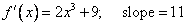d.3.a.b.c.d.4.a.b.c.d.5.a.b.c.d.6.a.b.c.d.7.a.b.c.d.8.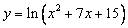a.b.c.d.### Quiz 2

1.  Find the formula for the slope of the following function:a.b.c.d.2   Find the formula for the slope of the following function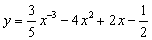a.b.c.d.3.  Find the formula for the slope of the following function:a.b.c.d.4.  Find the formula for the slope of the following functiona.b.c.d.5.  Find the formula for the slope of the following functiona.b.c.d.6.  Determine the slope and the rate of change of the slope of the following function when x=1:a.b.c.d.7.  Use the product rule and the exponent rule to find the derivative of the function: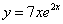a.b.c.d.8.  Although you can always simplify before using differentiation techniques, for practice use the exponent rule and the generalized power rule before simplifying to find the formula for the slope of the following function:a.b.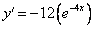c.d.9.  In the following function determine the value of the function, the slope, and the rate of change of the slope when x=-1.a.b.c.d.[Index]